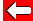# Using PercentagesHere's a question: Fraser scored 75% on a physics test. If there were 80 marks in total, how many marks did he lose?

Well, 75% means 75/100. So 25/100 would be wrong, which is 1 in every 4 marks - in other words one quarter.

One quarter of 80 is 20.

In general, we can solve questions like this by making the percentage equivalent to a decimal and multiplying it by the total.

e.g. 30% of 50 is 0.30 × 50 = 15.Go to next pageGo back a pageMaths Menu